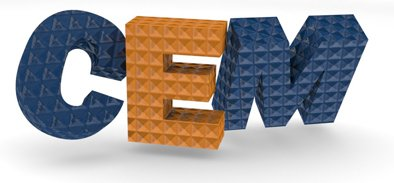Assignments

Homework Help

Course Topics

• (PDF) Lecture 0 – Rules and Procedures
• (PDF) (Video) Lecture 1 – Introduction to CEM
• (PDF) (Video) Lecture 2 – Maxwell’s Equations
• (PDF) (Video) Lecture 3 – Preliminary Topics in Computational Electromagnetics
• (PDF) (Video) Lecture 4 – Transfer Matrix Method
• (PDF) (Video) – Lecture 5a – Scattering Matricies for Semi-Analytical Methods
• (PDF) (Video)  Lecture 5b – Transfer Matrix Method Using Scattering Matrices
• (PDF)  Lecture 6 – Transfer Matrix Method Extras
• (PDF) (Video) Lecture 7 – Periodic Structures (Lecture 7 from EM21)
• (PDF) (Video) Lecture 8 – Diffraction Gratings and Plane Wave Spectrum
• (PDF) (Video) Lecture 9 – Perfectly Matched Layer
• (PDF) (Video) Lecture 10 – Finite Difference Method
• (PDF) (Video) Lecture 11 – Maxwell’s Equations On A Yee Grid
• (PDF) (Video) Lecture 12 – Finite Difference Analysis of Waveguides
• (PDF) (Video)  Lecture 13 – Finite Difference Frequency Domain (FDFD) Formulation
• (PDF) (Video)  Lecture 14 – Finite Difference Frequency Domain (FDFD) Implementation
• (PDF) (Video)  Lecture 15 – Finite Difference Frequency Domain (FDFD) Extras
• (PDF) (Video) Lecture 16 – Finite Difference Time Domain (FDTD)
• (PDF) (Video) Lecture 17 – Beam Propagation Method
• (PDF) (Video) Lecture 18 – Maxwell’s Equations In Fourier Space
• (PDF) (Video) Lecture 19 – Plane Wave Expansion Method
• (PDF)  Lecture 20 – Plane Wave Expansion Method Extras
• (Video) – MATLAB Session – Plane Wave Expansion Method (Photonic Band Diagrams)
• (Video) – MATLAB Session – Plane Wave Expansion Method (Isofrequency Contours)
• (PDF) (Video) Lecture 21 – Rigorous Coupled Wave Analysis Formulation
• (PDF) (Video) Lecture 22 – Rigorous Coupled Wave Analysis Implementation
• (PDF) (Video) Lecture 23 – Rigorous Coupled Wave Analysis Extras
• (PDF) (Video) Lecture 24 – Method of Lines
• (PDF) (Video) Lecture 25 – Slice Absorption Method
• (PDF) (Video) Lecture 26 – Introduction to Variational Methods
• Lecture 27 – Finite Element Method
• (PDF) Lecture 28 – Method of Moments for Thin Wires
• Lecture 29 — Method of Moments with RWG Edge Elements
• Lecture 30 — Spectral Domain Method
• (PDF) (Video) Lecture 31 – Optimization
• (PDF) Lecture 32 – Surface Propagation Methods

Other Resources

Electromagnetics Review

Topic Summaries

Animations and Visualizations